# Prediction and Validation of Pre-owned Car Price using Linear Regession Analysis¶

A multivariable linear regression was used to predict and validate the price of pre owned cars. In this post, you can find a step by step model implemntation, including data preprocessing, variable selection, model building, checking regression assumptions, taking corrective actions and testing the final model.

### Data Description¶

The data was obtained from the course material of Data mining in Engineering at Northeastern University, which was used to predict the price of pre owned Toyota Corolla using neural network. Here, I used a commonly used predictive model, linear regression, to predict the price of pre owned car. The data includes 38 variables and 1436 observations. The description of the variables is provided here.

### Multiple Linear Regression¶

Multiple linear regression model is used to fit a linear relationship between quantitative dependent variable (response variable) and multiple predictor variables. The model has the form:

$$\hat{Y}= \beta_0 + \beta_1x_1+ \beta_2x_2 +...+ \beta_nx_n$$$$\hat{Y}= X*\beta \hspace{0.4cm} (matrix \hspace{0.15cm} notation)$$

Where $\hat{Y}$ the predicted value of the response variable, $x_1$, $x_2$, ..., $x_n$ are predictor variables, $\beta_0$, $\beta_1$, ..., $\beta_n$ are unknown parameters to be predicted, and n is the number of predictor variables. Note that the model is called linear in the parameters ($\beta$'s) not in the $x$’s. Model parameters($\beta$s) are estimated in such a way that the difference between the actual (observed, Y) response values and the predicted values are minimized. Mathematically, minimizing the sum of squared residuals is provided as: $$min J(\beta) = min \sum\limits_{i=1}^m (Y_i - \hat{Y}_i)^2 = min \sum\limits_{i=1}^m (Y_i - (\beta_0 + \beta_1x_{i1} + \beta_2x_{i2} + ... + \beta_nx_{in}))^2$$

where $J(\beta)$ is the cost function, $Y_i$ is the $i^{th}$ observed response value and $m$ is total number of observations.

Optimization Algorithms:

Gradient decent: is an iterative method used to find the optimal values of $\beta$. It starts with some initial values of $\beta$ and simultaneously updates all $\beta$s using the following equation until it converges to minimum $J(\beta)$.

$\beta_j = \beta_j -\alpha \frac{\partial}{\partial\beta_j} J(\beta)$ , for all j from 0 to n(number of predictor variables)

$= \beta_j - 2\alpha \sum\limits_{i=1}^n (Y_i - (\beta_0 + \beta_1x_{i1} + \beta_2x_{i2} + ... + \beta_nx_{in}))^2 * x_{ij}$

Where $\alpha$ is the learning rate.

Normal equation: Unlike gradient decent, this method finds the optimum $\beta$ without iteration. $$\beta = (X^T X)^{-1}X^T Y$$

Where: $\beta$ is the vector of $\beta_0$, $\beta_1$, ..., $\beta_n$; $X$ is an $mx(n+1)$ matrix of the value of the predictor variables (one is added to handle the intercept term ($\beta_0$)); $X^T$ stands for the transpose of $X$, and $Y$ is the vector of the observed response values.

## Step 1: Data Exploration and Preprocessing¶

First, load all the necessary R packages that can be used to import, explore and alyze the data.

In :
# Important libraries
library(rJava)
library(xlsxjars)
library(xlsx)     # to read a file with .xlsx extension
library(car)      # to evaluate regression conditions
library(biglm)
library(leaps)    # to perform all subsets regression
library(stargazer)


The data was provided in excel file (xlsx format), and can be imported using the xlsx package as shown below, or can be converted into CSV file and then import into R using read.csv.

In :
Corolla<- read.xlsx('ToyotaCorolla.xlsx', sheetName="data")
#names(Corolla) # Variable names


You can list the variable names using names(mydata), and print the top n observations using head(mydata, n). The summary statistics of car price by each of the categorical variables is provided here. The overall mean and median car price were 10,731 and 9,900 respectively.

In :
#Summary statistics
stargazer(Corolla["Price"], type = "text", title="Summary statistics of Car Price",
digits=0, median=TRUE, iqr=TRUE, min.max=TRUE)

Summary statistics of Car Price
=====================================================================
Statistic   N    Mean  St. Dev.  Min  Pctl(25) Median Pctl(75)  Max
---------------------------------------------------------------------
Price     1,436 10,731  3,627   4,350  8,450   9,900   11,950  32,500
---------------------------------------------------------------------


Note that some of the categorical variables do have more than one categories and therefore a design matrix with dummy variables were created manually. It can also be done using the R function factor. For example, the variable Fuel_Type has three categories (Diesel, Petrol and CNG) and therefore we can create two dummy variables; i.e. if the fuel is diesel, a dummy variable "Fuel_Diesel" has a value of 1 otherwise 0. Similarly, a dummy variable "Fuel_Petrol" is 1 if it is petrol otherwise 0. CNG is taken as a reference.

In :
# Add dummy variables for fuel type  Diesel and Petrol by taking CNG as a base
Corolla$Fuel_Diesel= ifelse(Corolla$Fuel_Type=="Diesel", 1, 0)
Corolla$Fuel_Petrol=ifelse(Corolla$Fuel_Type=="Petrol", 1, 0)


### Split the Data into Training and Test Dataset¶

The data is divided into training and test data set to fit the regression model and to test the generalizablity of the model, respectively. Seventy percent of the observations was randomly picked as training data and the remaining 30% as test data.

In :
# Split the data into training and test data for validation purpose
training_size= round(nrow(Corolla)* 0.7)   # the size of training dataset (70%)
test_size= nrow(Corolla)-training_size     # the size of  test data set
training_sample= sample(nrow(Corolla), training_size,replace = FALSE, set.seed(1)) # select training data set

training_data= Corolla[training_sample, ]
test_data=Corolla[-training_sample, ]


### Variable Selection¶

A set of predictor variables among all list of variables were selected using all subset regression analysis. I prefer this method over stepwise regression variable selection method due to the fact that all subset methods checks possible combinations of variables.

#### All Subset Regression¶

All variables except model description, manufacturing month and year were included in the variable selection. The later two variables were excluded because they provide the same information as age of the car, which is already included in the model.

In :
#all subset regression plot
SubReg=regsubsets(Price ~., data=training_data[, -c(1,2,5,6,8,11,15)], nbest=2) # chooses the best two models for each subset size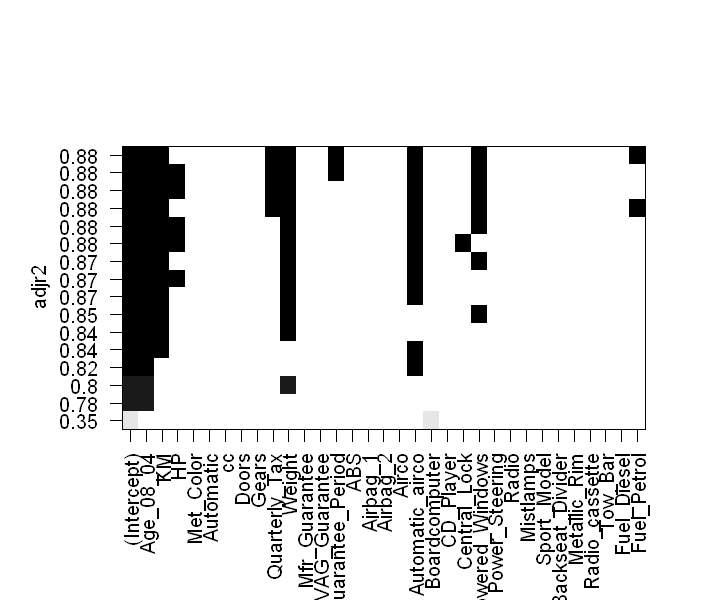You can read the above graph as follows: the rows correspond to a model, the shaded rectangle indicated the variables that are included in a given model, the labels in the x-axis are variables names, and the numbers in the y-axis are adjusted R-square. You can see from the above plot, starting from the bottom , a model with the intercept and Boardcomputer has adjusted R-square of 0.35 where as intercept and age has 0.78.

The following set of variables contains the dependent variable(price) and subset of predictor variables having the highest adjusted R-square from subset regression analysis.

In :
var= c("Price", "Age_08_04", "KM", "HP", "Quarterly_Tax", "Weight", "Automatic_airco"
,"Guarantee_Period" , "Powered_Windows")


## Step 2: Fitting the model¶

The model is fitted using ordinary least square method using the selected variables. As you can see the result, all coefficients are significantly different from zero at P<0.0001 level.

In :
model1= lm(Price~., data= training_data[var])
summary(model1)

Call:
lm(formula = Price ~ ., data = training_data[var])

Residuals:
Min      1Q  Median      3Q     Max
-5727.0  -742.5   -21.3   715.0  5033.4

Coefficients:
Estimate Std. Error t value Pr(>|t|)
(Intercept)       4.158e+03  1.146e+03   3.627 0.000301 ***
Age_08_04        -1.119e+02  2.995e+00 -37.375  < 2e-16 ***
KM               -1.972e-02  1.354e-03 -14.561  < 2e-16 ***
HP                2.102e+01  3.235e+00   6.497 1.29e-10 ***
Quarterly_Tax     6.682e+00  1.380e+00   4.841 1.50e-06 ***
Weight            1.011e+01  1.106e+00   9.144  < 2e-16 ***
Automatic_airco   2.717e+03  2.001e+02  13.579  < 2e-16 ***
Guarantee_Period  5.488e+01  1.345e+01   4.080 4.86e-05 ***
Powered_Windows   5.068e+02  8.488e+01   5.970 3.29e-09 ***
---
Signif. codes:  0 '***' 0.001 '**' 0.01 '*' 0.05 '.' 0.1 ' ' 1

Residual standard error: 1226 on 996 degrees of freedom
Multiple R-squared:  0.8815,	Adjusted R-squared:  0.8806
F-statistic: 926.3 on 8 and 996 DF,  p-value: < 2.2e-16


Model Interpretation: the model accounts 88.15% (see Multiple R-square)of the variance in car prices. The average error in predicting price using this model is 1226 Euro(see residual standard error).

In :
#Estimated coeffiecients and their 95% confidence interval
round(model1$coefficients, digits=2) # intercept and coefficents confint(model1) # 95% confidence interval of the intercept and the coefficients  (Intercept) 4157.81 Age_08_04 -111.93 KM -0.02 HP 21.02 Quarterly_Tax 6.68 Weight 10.11 Automatic_airco 2716.86 Guarantee_Period 54.88 Powered_Windows 506.79 2.5 %97.5 % (Intercept)1908.5166407.109 Age_08_04-117.8062-106.0527 KM-0.02238056-0.01706456 HP14.6699527.36684 Quarterly_Tax3.9736079.390801 Weight 7.94353912.284740 Automatic_airco2324.2273109.484 Guarantee_Period28.4870081.27653 Powered_Windows340.2167673.3633 Price = 4157.81 -111.93Age_08_04 - 0.02KM + 21.02HP + 6.68 Quarterly_Tax + 10.11 Weight + 2716.86Automatic_airco +54.88Guarantee_Period + 506.79Powered_Windows The model is interpreted in such a way that, for each increase in month of the age of the car , the selling price decreases by 111.93 Euro keeping other variables constant and so on. ## Step 3: Regression Diagnostics and corrective action¶ In order to use the fitted regression model, the following conditions have to be met: a) Normality: In the bivariate relations, for fixed values of the independent variable X, the dependent variable Y is normally distributed.If the dependent variable is normally distributed, the residual values should also be normally distributed. b) Constant variance(homoscedasticity): In the bivariate relations, for fixed values of the independent variable X, the variance of the dependent variable Y is constant. c) Linearity: All bivariate relations involved are linear. Thus, there should no be systematic relationship between the residual and the predicted values. d) Independence: the cases are independent of each other. e) Multicolinearity: little or no correlation between predictor variables. f) Influencial Observations: observations that influence parameter estimation. The statistical assumptions in regression analysis can be evaluated using plot() function from the base R or other functions from car package. Here, I used functions from car package to evaluate regression conditions. ### a) Normality¶ The function qqPlot() from car package is used to check the normality assumption. It plots the studentized residuals against a t- distribution with n(sample size) minus p(number of regression parameters including intercept) minus one degrees of freedom. As it is shown in the Q-Q plot, some values are out of the confidence envelop and far from the central line. In : qqPlot(model1, lables=row.names(No), simulate=TRUE, id.method="identify", main= "Q-Q Plot")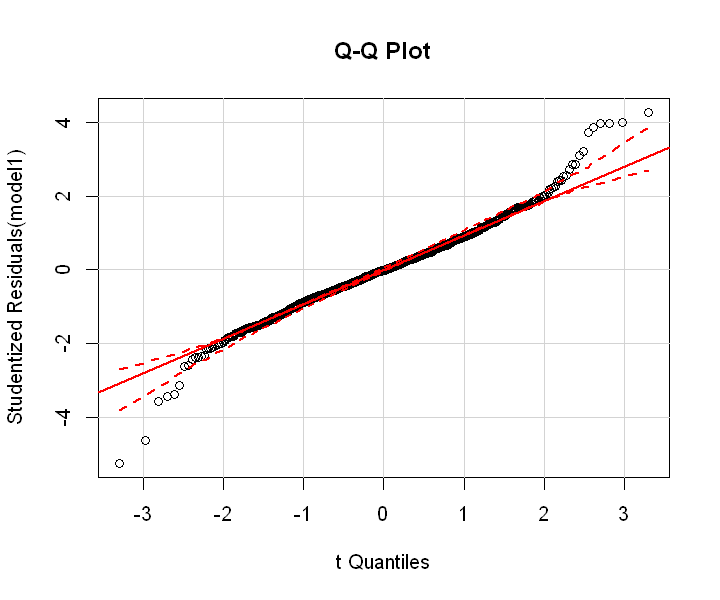The histogram and Kernel density curve, shown below, show the distribution of the error. It seams that the error closes to normal curve with the exception of some outliers. In : #Ploting studentized residual plot as histogram rs= rstudent(model1) hist(rs, breaks=20, freq=FALSE, xlab="Studentized Residuals", main="Distribution of Errors") curve(dnorm(x, mean=mean(rs), sd=sd(rs)), add=TRUE, col= "blue", lwd=2) lines(density(rs)$x, density(rs)$y, col= "red", lwd=2, lty=2) legend("topright", legend=c("Normal Curve", "Kernel Density Curve"), lty=1:2, col=c("blue", "red"), cex=.7)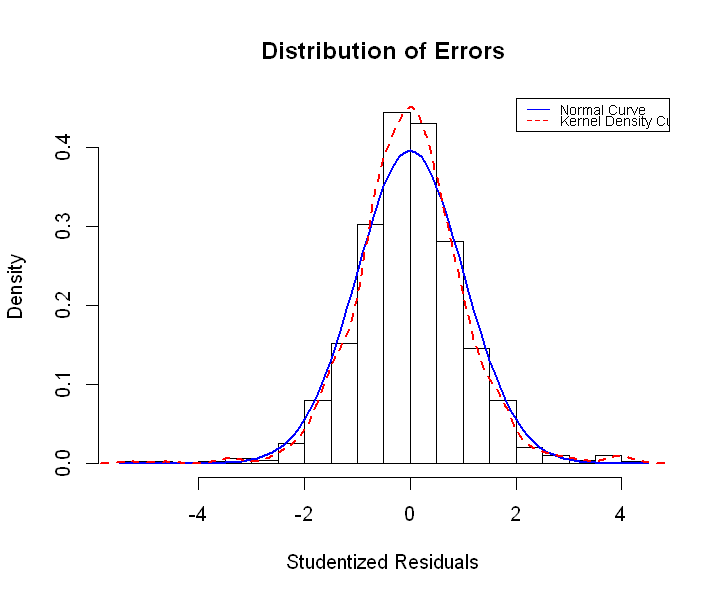Since normality assumption is primarly relevant to derive confidence interval for predictions and I have test data set to validate the model, the model can still be used without normality assumption is staisfied. Ingeneral, transformation of the response variable may help if normality assumption is violated. The function powerTransform() from car package can be used to estimate the transformation power of the response variable. ### b) Constant variance(Homoscedasticity)¶ The hypothesis of constant error variance against the alternative that error variance changes is tested and the result is obtained using the function ncvTest() from car package. The significant p value(8.585757e-19 ) indicates non constant error variance. In : ncvTest(model1)  Non-constant Variance Score Test Variance formula: ~ fitted.values Chisquare = 78.36037 Df = 1 p = 8.585757e-19  To stabilize the non-constant error variance, power transformation of 0.5213714(approximated as square root) is suggested by spread level plot. In : # creates scatter plot of the absolute standardized residuals vs fitted value spreadLevelPlot(model1)  Suggested power transformation: 0.5213714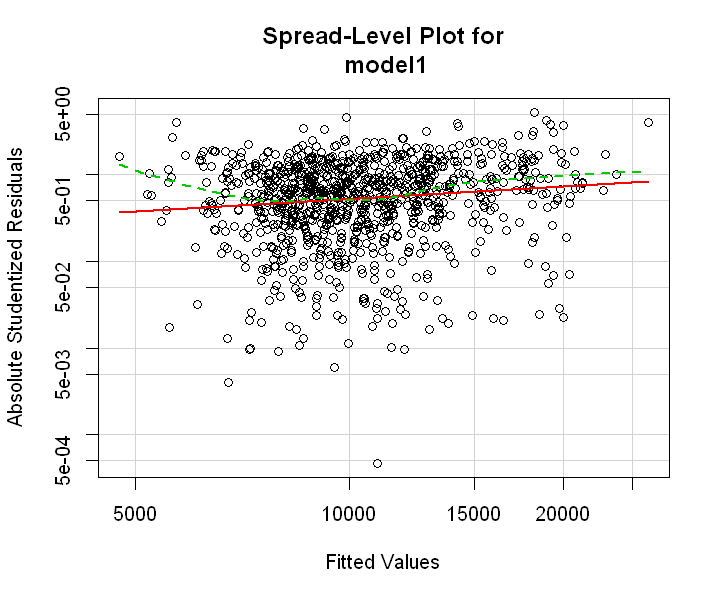### c) Linearity¶ Linearity assumption is checked by using crPlots() function from car package. The component plus residual plot (Partial residual plot) shows whether the relationships between the dependent and independent variables are linear. From the plot shown below, it is difficult to assume linear relationship between some independent and dependent variables. In : crPlots(model1, smooth=FALSE)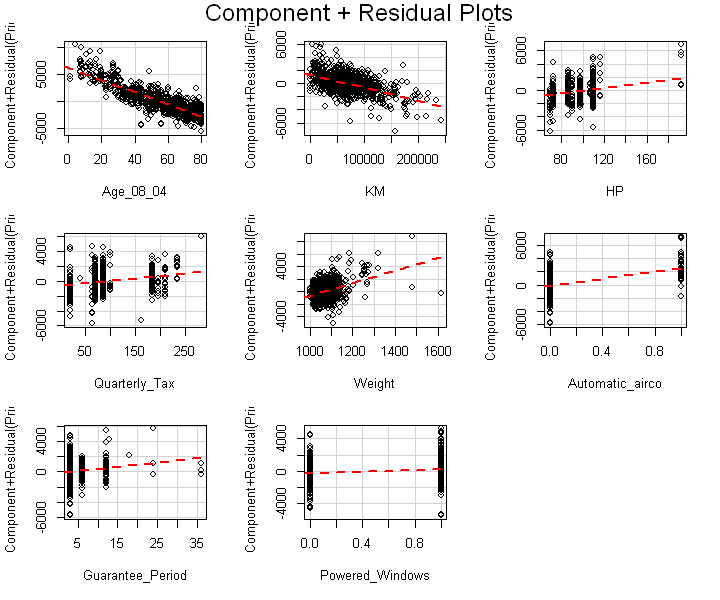Violation of linearity assumption can be corrected by power transformation of predictor variables. The function boxTidwell() from car package can be used to estimate the power of predictor variables. In this demonstration,it doesn't make sense to make power tranformation for variables that represent catagories such as Automatic_airco. Whereas, square root transformation of Age_08_04, the power of three transformation of HP and inverse of weight are estimated. Note that normality assumption and constant variance have to be met before transformation of predictor variable. Thus, square root transformation of the respons variable is done as suggested above. In : boxTidwell( sqrt(Price)~ Age_08_04 + KM + HP+ Quarterly_Tax + Weight,~ Guarantee_Period + Automatic_airco + Powered_Windows , data=training_data[var])   Score Statistic p-value MLE of lambda Age_08_04 5.769106 0.0000000 0.6758463 KM -4.083549 0.0000444 1.5243850 HP 2.164004 0.0304640 3.3549276 Quarterly_Tax -1.812064 0.0699763 0.0765634 Weight -2.063953 0.0390221 -1.3799086 iterations = 10  ### d) Independence of error¶ Generally, this assumption can be assesed by the knowledge about how the data were collected. But, we can also use Durbin-Watson test to detect correlated errors. The p-value is nonsignificant(>0.05) and thus the null hypothesis(defined as no autocorrelation) is accepted. Thus, the errors are independent. In : durbinWatsonTest(model1)   lag Autocorrelation D-W Statistic p-value 1 0.05718844 1.883975 0.08 Alternative hypothesis: rho != 0 ### e) Cheking multicollinearity¶ Multicollinearity between variables is also checked for this mode based on Variance Inflation Factor (VIF). I found that there is no multicollinearity problem. In : sqrt(vif(model1))>2 # if this is true there is multicollinearity problem  Age_08_04 FALSE KM FALSE HP FALSE Quarterly_Tax FALSE Weight FALSE Automatic_airco FALSE Guarantee_Period FALSE Powered_Windows FALSE ### f) Identifying influencing observation¶ Unusual observations such as outliers(having large positive or negative residuals), high leverage points(unusual combination of predictor values) and influential observations(influence on parameter estimation) are detected using different methods. I used the function outlierTest() to identify the outliers and influencePlot() to visualize outlier, leverage points and influencials in a single plot. In : outlierTest(model1) # list the significance of outliers   rstudent unadjusted p-value Bonferonni p 222 -5.261656 1.7483e-07 0.0001757 192 -4.623258 4.2747e-06 0.0042960 142 4.270805 2.1343e-05 0.0214500 In : # Combining outlier, leverage and influential plot together using influencial plot function influencePlot(model1, id.method="identify", main="Influence Plot", sub="Circle size is proportional to Cook's Distance")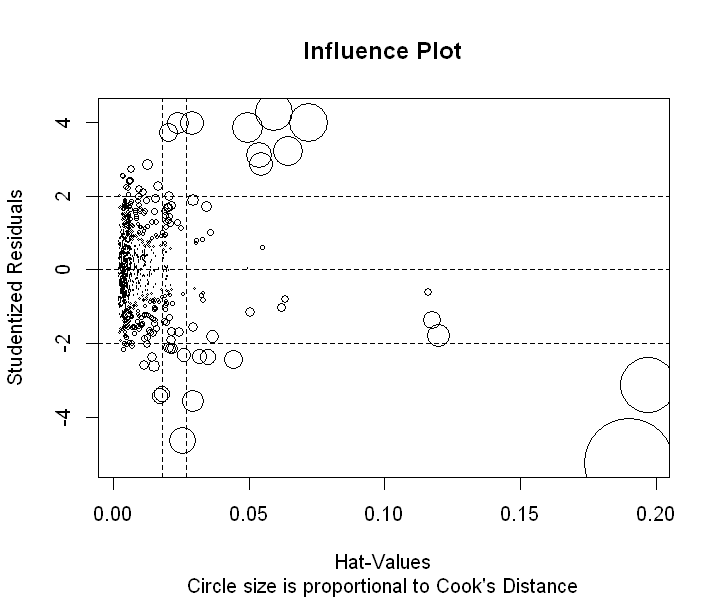Observations that have studentized residuals beyond -2 and 2 are outliers. Points to the right of the dotted vertical lines are leverage points and points that have large circle size are influencing observations. None of the observations are influencial as their Cook's distance is less than one. In : plot(model1, which=4, cook.levels=1)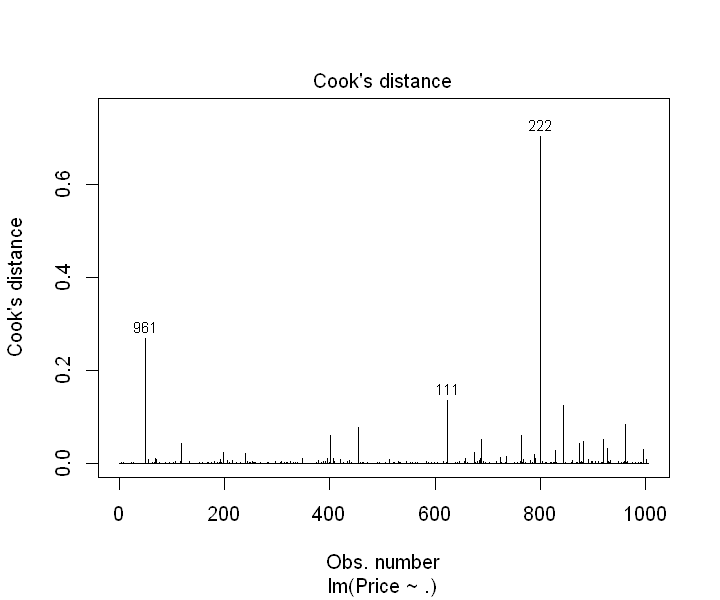## Step 4: Making the final model and testing its generalizablity¶ From regression diagnostic, we have seen whether regression assumptions are satisfied or not and corrective measures have been made. By combining all measures, a new model is proposed as: In : model2=lm(sqrt(Price)~sqrt(Age_08_04)+ KM + I(HP^3) + Quarterly_Tax + I(Weight^-1) + Automatic_airco + Powered_Windows ,data=training_data)  In : summary(model2)  Call: lm(formula = sqrt(Price) ~ sqrt(Age_08_04) + KM + I(HP^3) + Quarterly_Tax + I(Weight^-1) + Automatic_airco + Powered_Windows, data = training_data) Residuals: Min 1Q Median 3Q Max -31.4281 -3.0854 -0.1193 3.6969 24.9413 Coefficients: Estimate Std. Error t value Pr(>|t|) (Intercept) 1.894e+02 6.574e+00 28.813 < 2e-16 *** sqrt(Age_08_04) -6.875e+00 1.791e-01 -38.382 < 2e-16 *** KM -1.010e-04 6.091e-06 -16.575 < 2e-16 *** I(HP^3) 2.684e-06 3.603e-07 7.450 2.01e-13 *** Quarterly_Tax 2.990e-02 6.107e-03 4.896 1.14e-06 *** I(Weight^-1) -3.950e+04 6.812e+03 -5.798 9.01e-09 *** Automatic_airco 8.046e+00 9.056e-01 8.884 < 2e-16 *** Powered_Windows 2.354e+00 3.805e-01 6.185 9.05e-10 *** --- Signif. codes: 0 '***' 0.001 '**' 0.01 '*' 0.05 '.' 0.1 ' ' 1 Residual standard error: 5.564 on 997 degrees of freedom Multiple R-squared: 0.8777, Adjusted R-squared: 0.8769 F-statistic: 1022 on 7 and 997 DF, p-value: < 2.2e-16  This model is tested for all the assumptions and satisfies all. Despite the complexity of the model, this model is better than the original model as it has smaller Akaike Information Criterion(AIC) value. In : AIC(model1, model2)  dfAIC model1 10.0017156.38 model2 9.0006311.946 ### Testing the generalizablity of the model¶ The generalizablity of the model is tested using a new data set called test data. The model performs well for the new data set. One way to check its performance is by calculating the correlation between the predicted and actual values. They are highly correlated(0.959) In : pred_test= predict(model2,test_data) pred_price=pred_test^2 cat("Correlation = ", cor(test_data$Price,pred_price))

#Mean Absolute percentage error
cat("\nMean Absolute Percentage Error=", mean(abs(test_data$Price-pred_price)/test_data$Price))

Correlation =  0.9596535
Mean Absolute Percentage Error= 0.08292047
ObservedPredicted
313950.0015837.19
1120950.0022774.61
1421500.0021863.51
1522500.0021353.05
1622000.0022557.87
1722750.0021719.42

## Summary¶

Multiple linear regression is a commonly used predictive model for numerical response variable. This post demonstrates how multiple linear regression analysis can be done using R software from data processing all the way to testing the generalizablity of the selected model.